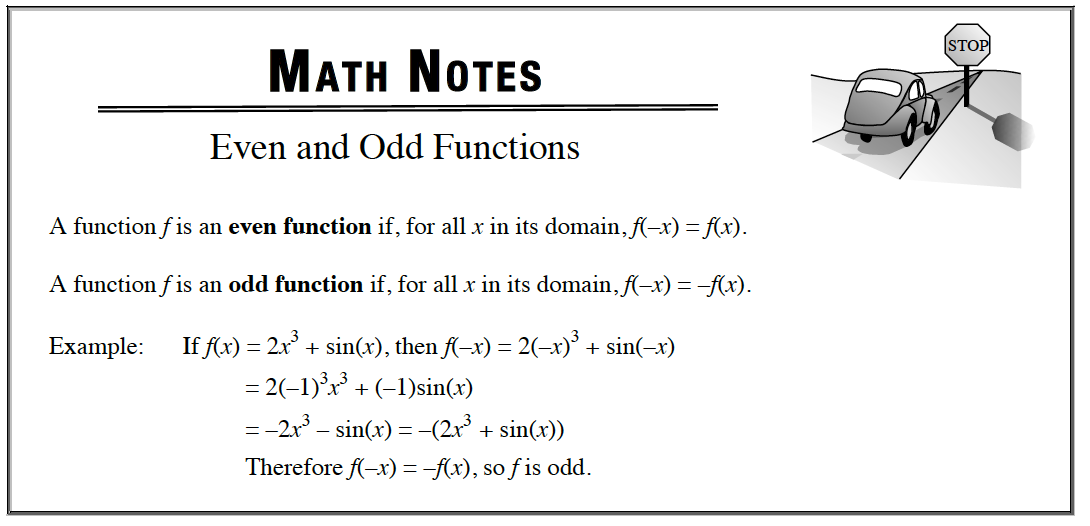### Home > APCALC > Chapter 1 > Lesson 1.2.5 > Problem1-82

1-82.

Are the following functions even, odd, or neither? Homework Help ✎

1. $y = x^{1/3}$

$\text{First, check if it is even:}$

$f(a)=a^{\frac{1}{3}}$

$f(-a)=(-a)^{\frac{1}{3}}=-a^{\frac{1}{3}}$

$\text{since }f(a) \neq f(-a),$

$f(x)\text{ IS NOT EVEN!}$

$\text{Next, check if it is odd:}$

$f(-a)=(-a)^{\frac{1}{3}}=-a^{\frac{1}{3}}$

$-f(a)=-a^{\frac{1}{3}}$

$\text{since }f(-a)= -f(a),{\ \ \ \ \ }f(x)\text{ IS ODD!}$

2. $y = −x^2 + 4$

Refer to the example in part (a). Be sure to simplify.

3. $y = x^3 + x^2 + 1$

Refer to the example in part (a). Be sure to simplify.